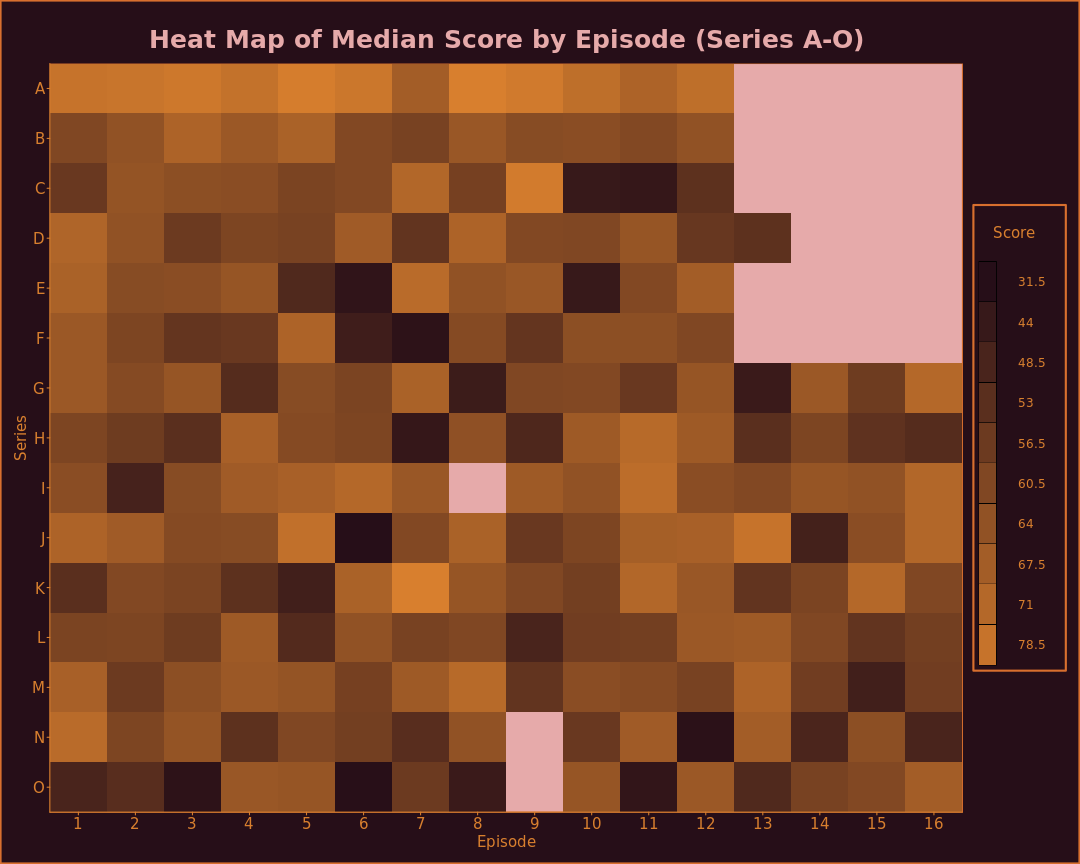# Quite Interesting (QI) Score Analysis

“Believe me, these [scores] are not invented, much as though people may believe it, the scores are rigorously and scientifically worked out.” -Stephen Fry

## Libraries

``````library(ggplot2)
``````

## Graph Functions and QI Theme

``````theme_qi <- function(){
theme_bw() %+replace% theme(
line = element_line(
colour="#260e18", size=0.5, linetype="solid", lineend="round"
),
text = element_text(
colour="#d87f2e",
family="Sans", face="plain", size=15, hjust=0.5, vjust=0.5,
angle=0, lineheight=2,
margin=margin(0.5, 0.5, 0.5, 0.5, "cm"), debug=FALSE
),
panel.border = element_blank(),
axis.line = element_line(colour="#d87f2e"),
axis.text = element_text(colour="#d87f2e"),
axis.ticks = element_line(colour="#d87f2e"),
panel.grid = element_line(colour="#d87f2e"),
plot.caption = element_text(
colour="#d87f2e", face="bold", size=25,
margin=margin(0.5, 0.5, 0.5, 0.5, "cm"), debug=FALSE
),
plot.subtitle = element_text(
colour="#d87f2e", face="bold", size=25,
margin=margin(0.5, 0.5, 0.5, 0.5, "cm"), debug=FALSE
),
plot.tag = element_text(
colour="#d87f2e", face="bold", size=25,
margin=margin(0.5, 0.5, 0.5, 0.5, "cm"), debug=FALSE
),
plot.title = element_text(
colour="#e6aaaa", face="bold", size=25,
margin=margin(0.5, 0.5, 0.5, 0.5, "cm"), debug=FALSE
),
legend.background = element_rect(
colour="#d86f2e", fill="#260e18", size=rel(2)
),
legend.key = element_rect(fill="#d87f2e"),
legend.margin = margin(0.2, 0.2, 0.2, 0.2, "cm"),
legend.spacing = unit(0, "cm"),
strip.background = element_rect(
colour="#d86f2e", fill="#260e18", size=rel(2)
),
panel.background = element_rect(
colour="#d86f2e", fill="#260e18", size=rel(3)
),
plot.background = element_rect(
colour="#d86f2e", fill="#260e18", size=rel(3)
),
plot.margin=margin(0.5, 0.5, 0.5, 0.5, "cm"),
panel.spacing=unit(0, "cm")
)
}
``````
``````qi_boxplot <- function(){
geom_boxplot(colour="#d87f2e", fill="#631d56")
}
``````
``````qi_errorbarh <- function(g, xmin, xmax){
g + geom_errorbarh(aes(xmin=xmin, xmax=xmax,
linetype="solid", size=0.5, colour="#d87f2e"
), show.legend=FALSE)
}
``````
``````qi_heatmap <- function(data){
data\$Series <- factor(data\$Series,
levels=rev(unique(as.character(data\$Series)))
)
data\$Score <- as.factor(data\$Score)
data_nlev <- nlevels(data\$Score)
qi_palette <- colorRampPalette(c("#260e18", "#d87f2e"))(data_nlev)

g <- ggplot(data, aes(x=Episode, y=Series, fill=Score))
g <- g + geom_tile() + scale_fill_manual(
values=qi_palette,
breaks=c("NA", levels(data\$Score)[seq(1, data_nlev, by=data_nlev/10)])
)

g <- g + scale_x_discrete(expand=c(0,0)) + scale_y_discrete(expand=c(0,0))

g <- g + theme_qi() %+replace% theme(
panel.grid = element_line(linetype=0),
panel.background = element_rect(
colour="#d86f2e", fill="#e6aaaa", size=rel(1)
)
)

g
}
``````
``````qi_line <- function(){
geom_line(colour="#d87f2e", size=1.5)
}
``````
``````qi_point <- function(){
geom_point(colour="#d87f2e", size=1.7)
}
``````
``````rotate_x <- theme_qi() %+replace% theme(
axis.text.x = element_text(angle=90, size=15, colour = "#d87f2e")
)
``````

``````qi_scores <- read.csv("qi-scores.csv")
``````

## Data Remediation and Explanation

Ranking is done via modified competition ranking.

Scores are given based on quizmaster score definition first, then the scores are ranked as such. These rankings overrule the quizmaster, so If in the instance a a tied rank is given in another means (contestant A is “first”, contestant B and C tied for “second”), these calculated rankings overrule the quizmaster. The reason for this is that the QI ranking as provided by the quizmaster is never consistant, sometimes a tie produces competition ranking, sometimes modified competition ranking.

All other specific changes to scores and exceptions to these rules are explained below.

#### S01E00 - Pilot

Scores are done in a completely different manner and are not comparable, so they are ignored and omitted for analysis. Rankings are preserved and kept.

``````qi_scores[
qi_scores\$Series == "A" & qi_scores\$Episode == 0,
c("Contestant", "Score")
]
``````
``````##           Contestant Score
## 1        Alan Davies   118
## 2        Bill Bailey   132
## 3       Eddie Izzard   131
## 4 Kit Hesketh-Harvey   125
``````
``````qi_scores\$Score[qi_scores\$Series == "A" & qi_scores\$Episode == 0] <- NA
``````

#### S01E06 - Antidotes

Danny Baker received first place, but no score was given by Fry.

#### S04E08 - Descendants

Scores were given in millions, which needs to be adjusted for comparative purposes.

``````qi_scores\$Score[qi_scores\$Series == "D" & qi_scores\$Episode == 8] <- qi_scores\$Score[qi_scores\$Series == "D" & qi_scores\$Episode == 8]/1000000
``````

#### S06E04 - Fight or Flight

Pam Ayres received first place, but no score was given by Fry.

#### S07E02 - G-Animals

John Hodgman received first place, but no score was given by Fry.

#### S08E07 - Horrible

Chris Addison received second place with 13.8, while first place was given to Dara O Briain who had 2 points. This was adjusted in the dataset to be -13.8 as it was likely Fry misspeaking since third place had -33 (Sean Lock) and scores were said in first to last order.

#### S09E08 - Inequality

“And so we come to the scores. Well now, these are very interesting, and it would be very unfair of me not to share them with you… So, that's all from Sandi, Henning, Clive, Alan, and me, because as William Goldman said, 'Life isn't fair, it's just fairer than death.' That's all. Goodnight.” -Stephen Fry

#### S10E08 - Jumble

Stephen Fry awarded himself 52! (factorial) points for explaining how complex a deck of cards is and producing a “world first” by shuffling a deck of cards. This was recorded in the data as Stephen Fry getting first place and everyone else shifting down, however the score is not recorded since it's a huge number.

#### S11E08 - Keys

Tim Minchin and Alan Davies received third and fourth places respectively, but no scores were given by Fry.

#### S12E07 - Lethal

Alan Davies received third place, but no score was given by Fry.

#### S13E03 - M-Places

Sami Shah, Alan Davies, and Sue Perkins received second, third, and fourth places respectively, but no scores were given by Fry.

#### S14E09 - Noel

For Christmas, everybody won and no scores were given out. This would produce a tie for fourth given the ranking system being employed, so this data is not used.

#### S14E14 - N-Numbers

Despite receiving -26 points, which would have given her third place based on the scores, Sarah Millican received first place due to Sandi Toksvig missing an event to become the new quizmaster. This bit of cronyism was reflected in the data.

#### S15E09 - O Christmas

For Christmas, everybody won and no scores were given out. This would produce a tie for fourth given the ranking system being employed, so this data is not used.

#### S15E12 - Occult

Russell Brand received third place, but no score was given by Toksvig.

#### Victoria Coren Mitchell

For record keeping purposes, Victoria Coren Mitchell's early name is kept as “Victoria Coren” in the data, yet after she married David Mitchell the name change shouldn't change her record, so altering that to fix this discrepency.

``````qi_scores\$Contestant[qi_scores\$Contestant == "Victoria Coren"] <- "Victoria Coren Mitchell"
``````

## Data Preparation

### Calculate Rankings

``````qi_scores <- qi_scores[
order(qi_scores\$Series, qi_scores\$Episode, -as.numeric(qi_scores\$Score)),
]

needs_ranking <- is.na(qi_scores\$Ranking) & !is.na(qi_scores\$Score)
split_factor <- as.factor(paste0(
qi_scores\$Series[needs_ranking], qi_scores\$Episode[needs_ranking]
))

qi_scores\$Ranking[needs_ranking] <- unsplit(
lapply(
split(
-as.numeric(qi_scores\$Score[needs_ranking]),
split_factor
), FUN=function(x){
rank(x, ties.method="max");
}
), split_factor
)
``````

### Remove Outliers

Only one, Alan Davies' 689.66 in episode K-7 produces some weird and hard to read graphs.

``````qi_scores_noout <- qi_scores[!(qi_scores\$Series == "K" & qi_scores\$Episode == 7 & qi_scores\$Contestant == "Alan Davies"),]
``````

### Calculate Frequency of Appearance

``````freq_appearance <- aggregate(Score ~ Contestant, data=qi_scores, FUN=length)
names(freq_appearance) <- c("Contestant", "Appearances")
``````

### Common Contestants

Common contestants are defined as those contestants that have appeared in the show five or more times.

``````common_scores <- qi_scores_noout[qi_scores_noout\$Contestant %in% freq_appearance\$Contestant[freq_appearance\$Appearances >= 5],]
``````

### Common Contestant's Appearances by Date (Series A-O)

``````common_scores\$Episode <- as.factor(common_scores\$Episode)
common_scores\$Date.Aired <- as.POSIXct(common_scores\$Date.Aired)
min_ag <- aggregate(Date.Aired ~ Contestant,
data=common_scores,
FUN=function(x){ min(x, na.rm=TRUE); }
)
names(min_ag) <- c("Contestant", "Min.Date")
max_ag <- aggregate(Date.Aired ~ Contestant,
data=common_scores,
FUN=function(x){ max(x, na.rm=TRUE); }
)
names(max_ag) <- c("Contestant", "Max.Date")
common_appearances_range <- merge(min_ag, max_ag)
names(common_appearances_range) <- c("Contestant", "Date", "Max.Date")
common_appearances_range\$Contestant <- factor(
common_appearances_range\$Contestant,
levels=rev(levels(common_appearances_range\$Contestant))
)
``````
``````g <- ggplot(common_appearances_range, aes(x=Date, y=Contestant))
g <- qi_errorbarh(g,
common_appearances_range\$Date,
common_appearances_range\$Max.Date
)
g <- g + ggtitle("Common Contestant's Appearances by Date (Series A-O)")
g <- g + labs(x=NULL, y=NULL)
g <- g + theme_qi()
g
``````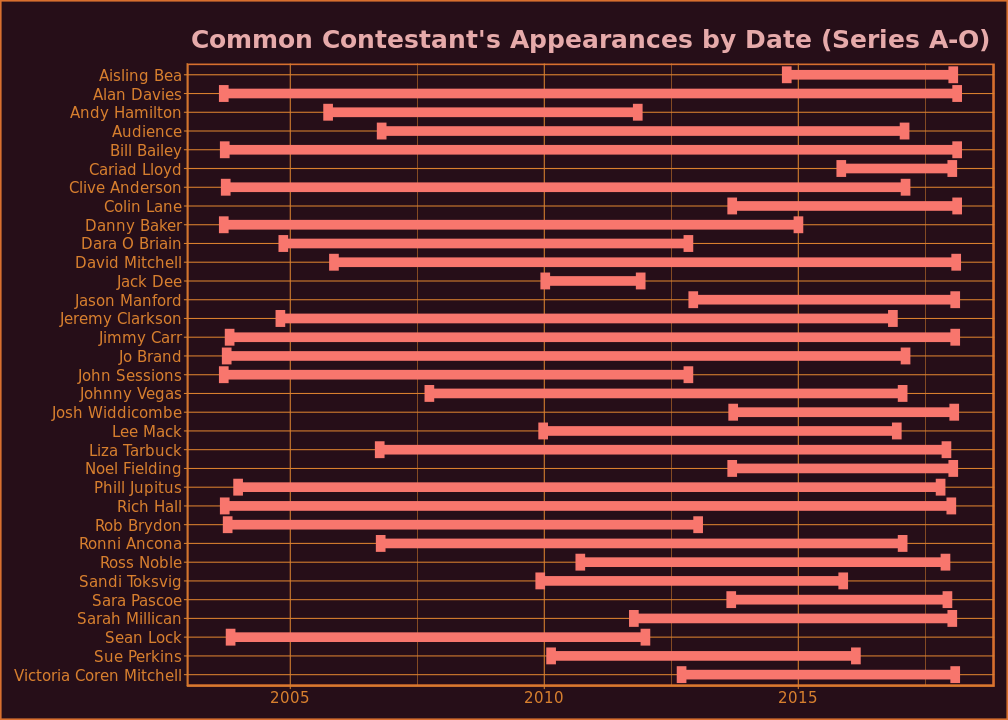## Mean Rankings

``````mean_ranks <- aggregate(Ranking ~ Contestant, data=common_scores, FUN=mean)
names(mean_ranks) <- c("Contestant", "Mean.Ranking")
mean_ranks <- mean_ranks[order(mean_ranks\$Mean.Ranking),]
rownames(mean_ranks) <- seq(1:nrow(mean_ranks))
mean_ranks
``````
``````##                 Contestant Mean.Ranking
## 1             Cariad Lloyd     1.428571
## 2            Sandi Toksvig     1.533333
## 3  Victoria Coren Mitchell     1.600000
## 4               Ross Noble     1.833333
## 5                Rich Hall     1.880000
## 6              Aisling Bea     2.000000
## 7              Danny Baker     2.125000
## 8           Clive Anderson     2.133333
## 9            Andy Hamilton     2.142857
## 10          David Mitchell     2.250000
## 11           Dara O Briain     2.285714
## 12            Ronni Ancona     2.285714
## 13            Johnny Vegas     2.300000
## 14           John Sessions     2.400000
## 15           Phill Jupitus     2.421053
## 16                Jack Dee     2.500000
## 17           Noel Fielding     2.500000
## 18             Sara Pascoe     2.500000
## 19              Rob Brydon     2.533333
## 20             Bill Bailey     2.558140
## 21         Jeremy Clarkson     2.571429
## 22            Liza Tarbuck     2.600000
## 23          Sarah Millican     2.666667
## 24                Jo Brand     2.675676
## 25               Sean Lock     2.740741
## 26              Jimmy Carr     2.771429
## 27           Jason Manford     2.800000
## 28              Colin Lane     2.833333
## 29                Audience     3.000000
## 30         Josh Widdicombe     3.000000
## 31             Alan Davies     3.158879
## 32                Lee Mack     3.200000
## 33             Sue Perkins     3.400000
``````

## Score Analysis and Graphs

And now onto the scores, and my how fascinating they are.

### Median Scores

``````median_scores <- aggregate(Score ~ Contestant, data=common_scores, FUN=median)
median_scores <- median_scores[order(-median_scores\$Score),]
rownames(median_scores) <- seq(1:nrow(median_scores))
median_scores
``````
``````##                 Contestant Score
## 1            Sandi Toksvig   6.0
## 2             Ronni Ancona   5.0
## 3             Cariad Lloyd   4.0
## 4                Rich Hall   3.0
## 5  Victoria Coren Mitchell   3.0
## 6            Andy Hamilton   2.0
## 7            Dara O Briain   2.0
## 8             Johnny Vegas   1.5
## 9           Clive Anderson   0.0
## 10              Ross Noble  -0.5
## 11                Audience  -2.5
## 12                Jack Dee  -3.5
## 13           John Sessions  -3.5
## 14           Noel Fielding  -3.5
## 15           Phill Jupitus  -4.0
## 16         Jeremy Clarkson  -4.5
## 17             Bill Bailey  -5.0
## 18          David Mitchell  -5.0
## 19             Aisling Bea  -6.0
## 20              Jimmy Carr  -6.0
## 21              Rob Brydon  -6.0
## 22             Sara Pascoe  -6.5
## 23           Jason Manford  -7.0
## 24               Sean Lock  -7.0
## 25                Jo Brand  -8.0
## 26            Liza Tarbuck  -8.0
## 27              Colin Lane  -9.0
## 28         Josh Widdicombe -10.0
## 29          Sarah Millican -10.0
## 30             Danny Baker -13.0
## 31             Alan Davies -14.0
## 32             Sue Perkins -15.5
## 33                Lee Mack -18.0
``````

### Alan Davies' Scores

``````alan_scores <- qi_scores[qi_scores_noout\$Contestant == "Alan Davies",]
alan_scores\$Episode.Number <- seq(1:nrow(alan_scores))

g <- ggplot(alan_scores, aes(x=Episode.Number, y=Score))
g <- g + ggtitle("Alan Davies' Scores (Series A-O)")
g <- g + qi_point() + qi_line() + theme_qi()
g
``````
``````## Warning: Removed 2 rows containing missing values (geom_point).
``````
``````## Warning: Removed 1 rows containing missing values (geom_path).
``````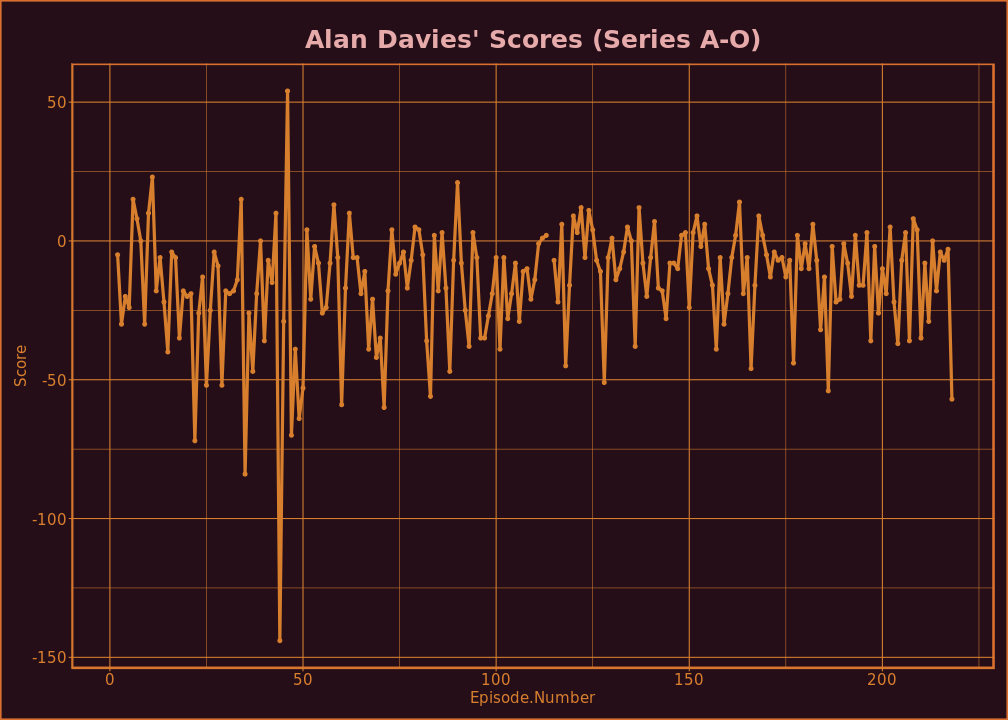### Common Contestants Score Distribution

``````g <- ggplot(common_scores, aes(x=Contestant, y=Score))
g <- g + ggtitle("Common Contestants Score Distribution Comparison (Series A-O)")
g <- g + qi_boxplot() + theme_qi() + rotate_x
g
``````
``````## Warning: Removed 13 rows containing non-finite values (stat_boxplot).
``````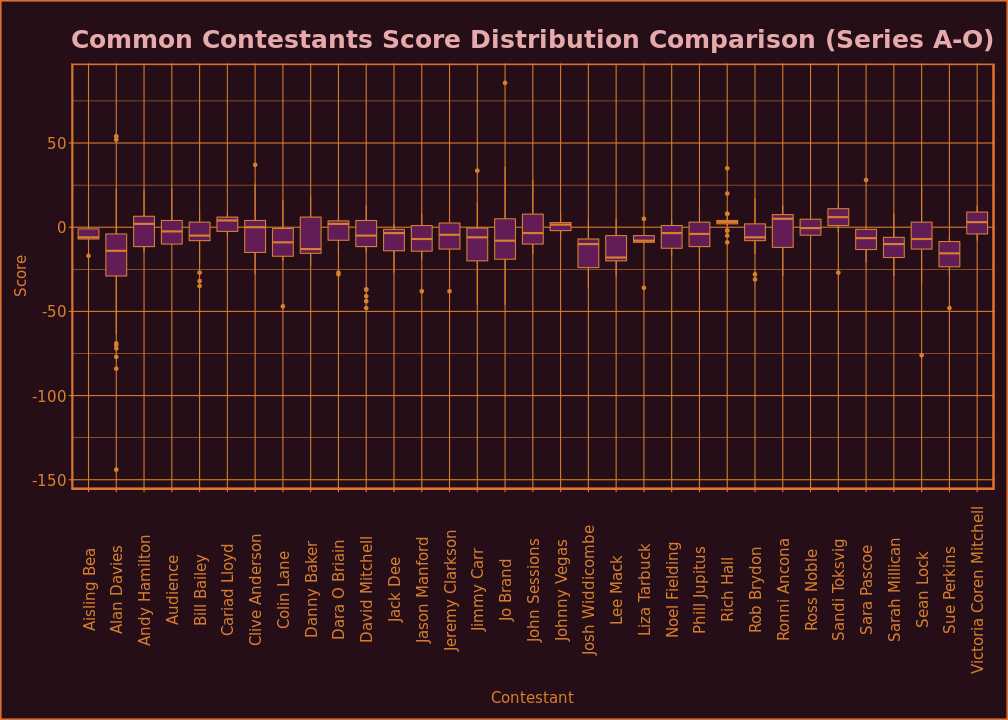### Heat Maps by Episode

#### Prep

``````qi_heatscores <- qi_scores_noout
qi_heatscores\$Episode <- as.factor(qi_heatscores\$Episode)
qi_heatscores\$Score <- as.factor(qi_heatscores\$Score)
``````

All episodes have their scores summed up. It is possible to potentially infer the approximate number of klaxons per show based on this map.

``````heat_sum <- aggregate(
as.numeric(Score) ~ Episode + Series,
data=qi_heatscores, FUN=sum
)
names(heat_sum) <- c("Episode", "Series", "Score")
g <- qi_heatmap(heat_sum)
g <- g + ggtitle("Heat Map of Total Score by Episode (Series A-O)")
g
``````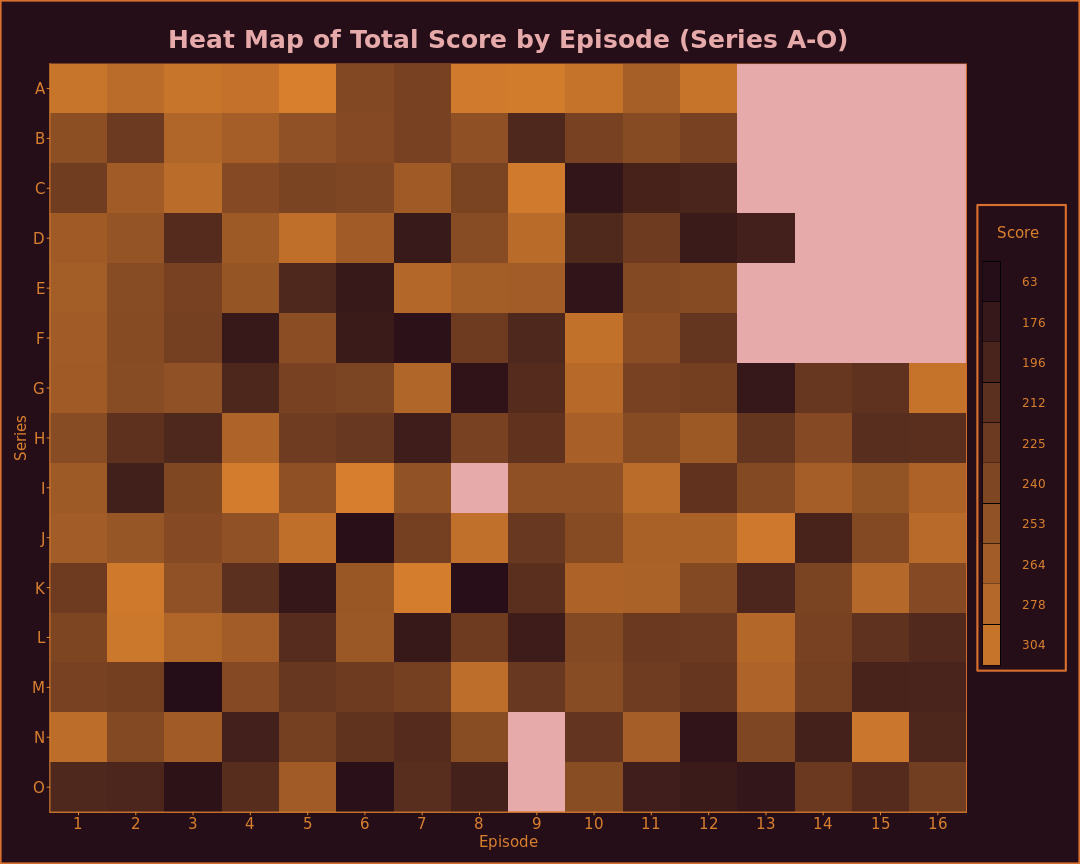All episodes use median scores.

``````heat_median <- aggregate(
as.numeric(Score) ~ Episode + Series,
data=qi_heatscores, FUN=median
)
names(heat_median) <- c("Episode", "Series", "Score")
g <- qi_heatmap(heat_median)
g <- g + ggtitle("Heat Map of Median Score by Episode (Series A-O)")
g
``````